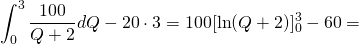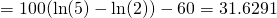# Solution assignment 04 Area and volume

### Assignment 4

Calculate the area which is enclosed by the lines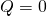and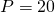and the graph of the function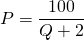.
This assignment is relevant for the calculation of the Producer Surplus (see Bradley T. en Patton P., Essential Mathematics for Economics and Business, 2e ed., John Wiley, p. 416).

### Solution

The intersection point of the graph of the functionand the lineis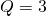. Thus the area equals the area enclosed by the graph of,and, minus the area of the rectangle through the points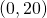and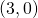.
Then we get: